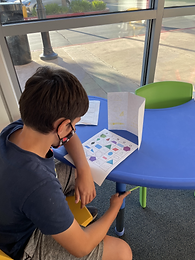## Ms. Caitlyn

### Target 1​

###### Lesson Type:

Continuation

Number Operation

:

Computation

Solve multi-step word problems within 1,000,000 (addition, subtraction, multiplication, and division).

###### 1:

Identify the correct arithmetic processes based on the information presented in word problems.

###### 2:

Find the key words in word problems that indicate the correct arithmetic process to use: Addition: add, altogether, both, combined, in all, plus, sum, total; Subtraction: difference, fewer, how many/much more, left, less, minus, remains; Multiplication: times, product, each, twice; and Division: how many in each, divided by, quotient, goes into, split evenly.

###### 3:

Find and use the needed information in a word problem to solve.

5th

###### Vocabulary:

Key Numbers, Math Action Words, Relevant Information, Operation, Expression

Activities:

Students continued to utilize the CUBES Method to discern key from relevant information.

Students solved a set of word problems independently, both with and without the CUBES Method to compare fluency and efficiency.### Home Exploration

###### Guiding Questions:## Absent Students:

### Target 2

:

###### 1:

Define each type of triangle (scalene, isosceles, equilateral, obtuse, acute, and right).

###### 2:

Identify triangles by their defining attributes (number of sides, number of vertices, vertices angle measurements, and side lengths).

###### 3:

Define each type of quadrilateral (square, rectangle, parallelogram, rhombus, and trapezoid).

5th

###### Vocabulary:

Quadrilateral, Triangle, Scalene, Isosceles, Equilateral, Sides

Activities:

Students created a brochure about classifying triangles by their sides (scalene, isosceles, and equilateral).### Home Exploration

###### Guiding Questions:### Target 3

:

###### Vocabulary:

Activities:### Home Exploration